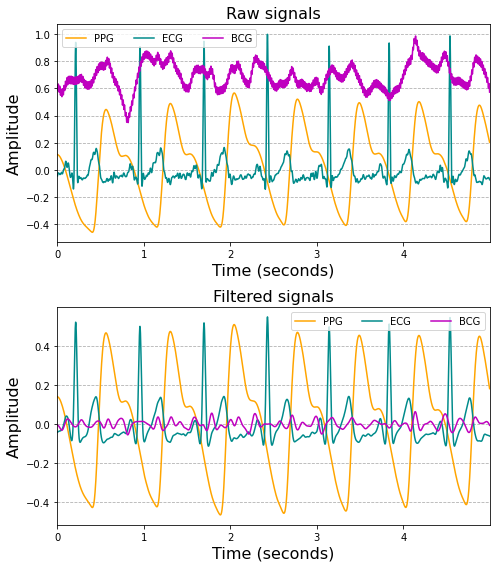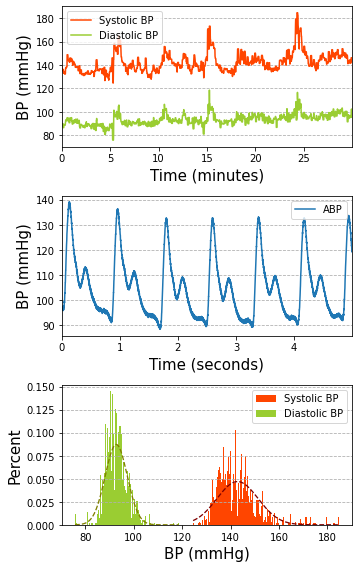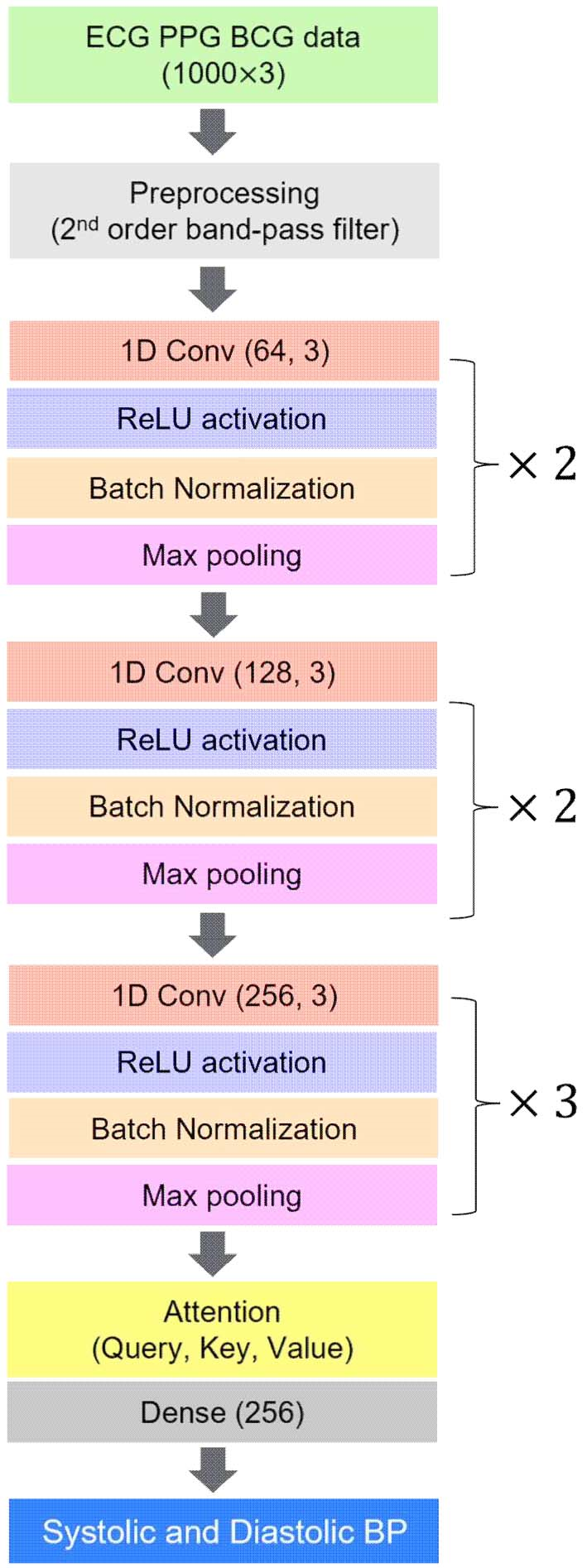Mobile QR Code1. (Department of Computer Engineering, Kwangwoon University / Seoul, Korea youjungseo0317@gmail.com, ghks4098@naver.com, unangsunarya2040@gmail.com, parkcheolsoo@kw.ac.kr )
2. (R&D Department, TVSTORM, Inc. / Seoul, Korea kwangkeelee@gmail.com)

Luong attention, Blood pressure, 1D convolutional neural network, Attention mechanism

## 1. Introduction

Biopotentials such as electrocardiogram (ECG), photoplethysmogram (PPG), and ballistocardiogram (BCG) signals are widely used for cuffless blood pressure (BP) estimation due to their non-invasive approach . Biosignals are used to calculate pulse transit time (PTT)—the time taken by a pulse wave between two arterial sites—which has an inverse correlation with BP .

Several deep neural network (DNNs) models have been proposed to predict PTT-based blood pressure [3-6]. In particular, the recurrent neural network (RNNs) models is the most widely used architecture, since it could extract the sequential information from time series data . However, one of the major obstacles of the RNNs approach is a long-term dependency when processing the sequential data. As the layers in networks become deeper, initial weights could not be considered due to the vanishing gradient problem . Additionally, the training time for RNNs increases significantly when training data have long sequences . Thus, an RNNs might not be a suitable model for edge devices like mobile phones or smart watches owing to its high computational costs.

Therefore, this paper investigates an attention mechanism particularly known as Luong attention to estimate the BP using the data of three channels: ECG, PPG, and BCG signals. The proposed algorithm comprises four layers of a 1D convolutional neural network (CNNs) followed by a self-attention algorithm to extract correlations among segments of the input data sequences. The attention mechanism is faster than the RNNs due to its parallel computations for all input data . Moreover, the attention mechanism is explainable, making it more interpretable than conventional DNNs.

The remaining sections cover the data acquisition process, a demonstration of the algorithm, and the performance results.

## 2. Data Acquisition

The data used for model training and testing are as follows. Five subjects participated in the experiment, and 30-minute long data from ECG and PPG signals were recorded using a biosignal amplifier (Biopac MP36, BIOPAC Systems Inc., Goleta, CA, USA). A BCG signal was simultaneously measured using a data acquisition module (NI 6225, National Instrument Corp, Austin, TX, USA). At the same time, continuous ABP was measured using a beat-to-beat BP monitor (Finometer PRO, Netherlands).

This experiment was approved by the Institutional Review Board of Kwangwoon University (IRB No.7001546-20200823-HR(SB)-008-07). During the experiment, subjects were introduced to a cold pressor condition three times, where the subject’s right foot was put into cold water for one minute to elevate blood pressure.

### 2.1 Data Preprocessing

After the data were obtained at the sampling frequency (1KHz), band-pass filtering was performed to remove noise and baseline wandering. Fig. 1 illustrates the signals before and after band-pass filtering. Table 1 shows the cutoff frequency of the band-pass filter for each signal. For the input data, five-second windows with corresponding SBP and DBP were used to train and test the model. Fig. 2 displays the continuous BP values utilized for labels to train the model. Outlier BP values beyond the range of the mean ${\pm}$1.96 standard deviation (SD) were removed from the dataset.

##### Fig. 1. The preprocessing of input signals (Top: raw signals; Bottom: filtered signals).##### Fig. 2. Continuous BP used as labels to train and test the model (Top: continuous BP for 30 mins; Middle: arterial BP from Finometer; Bottom: distribution of BP).##### Table 1. Cutoff frequencies of band-pass filtered input.
 Signal High-pass filter Low-pass filter ECG 0.5 Hz 35 Hz PPG 4 Hz 15 Hz BCG 0.5 Hz 15 Hz

### 2.2 Deep Learning Architecture

The proposed model to estimate BP consists of a 1D CNN and the Luong attention mechanism. The overall architecture is illustrated in Fig. 3. First, the preprocessed input data are fed into the 1D CNN for feature extraction. The dimensions of the initial data are 1000 $\times 3$, and the CNN layers are composed of 64, 128, and 256 layers, followed by batch normalization and max pooling to downsample the features.

##### Fig. 3. The overall architecture of the proposed DNN model.### 2.3 Attention Mechanism

After the CNN module, the output dimensions are 625~$\times 256$, where 625 is the length of the segments, and 256 is the number of features. The output is fed through the attention layer to emphasize the significant features by calculating the attention scores.

By calculating the attention weights between the input feature state and the output feature state, the attention mechanism discovers which feature contributes the most to estimating the answer . The attention scores are calculated as follows: a set of query, key, and value vectors is generated, where the query is known as the hidden state of an encoder, and the key and value are the hidden states of the decoder at time $t$. In this paper, the query, key, and value vectors come from the output of the previous CNN layer.

Eq. (1) shows the overall attention mechanism. First, alignment score $a_{t}$ at time $t$ is calculated from the dot product of query vector $h_{t}$ and key vectors $h_{s}^{T}$. Next, the alignment score is multiplied by value vector $h_{t}$ to generate a weighted sum as context vector $c_{t}$. Finally, the context vector is concatenated with the input of the decoder, $h_{t}$, and is fed through the $tanh$ function to predict the answer, $\overset{˜}{h}_{t}$.

##### (1)
\begin{gathered} a_{t}(s)=\operatorname{align}\left[h_{s}^{T}, h_{t}\right] \\ c_{t}=\sum a_{t} h_{t} \\ \tilde{h}_{t}=\tanh \left(W_{c}\left[c_{t}^{T}, h_{t}\right]\right) \end{gathered}

## 3. Results

The performance of the model was validated based on mean absolute error (MAE), root mean square error (RMSE), and the $R^{2}$ coefficient. The metrics are shown in Eqs. (2) and (3).

In Eqs. (2) and (3), the evaluation metrics are described in which $y$ denotes ground truth, and $\hat{y}$ is the reference BP. MAE and RMSE represent the difference between the predicted values and ground truth. $R^{2}$ provides the correlation coefficient between the prediction and the reference BP:

##### (2)
$MAE=~ \frac{1}{n}\left| y-\hat{y}\right| ^{2} \\$
##### (3)
$RMSE=\sqrt{\sum _{i=1}^{n}\frac{\left| y-\hat{y}\right| ^{2}}{n}}~$

Table 2 shows that the MAE was 3.299${\pm}$2.419 for SBP and 2.69${\pm}$1.821 for DBP. The results for MAE and RMSE outperformed models that connect the CNN, RNN, and attention algorithms .

##### Table 2. The performance results.
 BP MAE RMSE SBP 3.299±2.419 4.091 DBP 2.69±1.821 3.249

### 3.1 Discussion

The proposed model is a DNN-based cuffless blood pressure estimation algorithm using ECG, PPG, and BCG signals. In particular, the attention mechanism is mainly applied to learn sequential information from input data where no recurrent neural network is used.

The attention mechanism is preferable to the conventional RNN, since it requires less computational power and eliminates the vanishing gradient issue. The attention mechanism applied in this study is also called multiplicative attention, suggesting a different approach from the additive Bahdanau attention . The main contribution of this paper is a noble DNN architecture with the attention mechanism, which (owing to its light weight) could be equipped in a wearable device to monitor BP in patients’ daily lives.

## 4. Conclusion

In this paper, we proposed a cuffless BP estimation model using an attention mechanism that does not require an RNN and that yields a highly accurate BP prediction. This approach could be an alternative to the conventional cuff-based BP monitoring model, and is more accessible and comfortable when applied on a daily basis.

### ACKNOWLEDGMENTS

This work was supported by an Institute of Information & Communications Technology Planning & Evaluation (IITP) grant funded by the Korean government (MSIT). (No. 2021-0-00900, Adaptive Federated Learning in Dynamic Heterogeneous Environment).

### REFERENCES

1
Wang R., Jia W., Mao Z. H., Sclabassi R. J., Sun M., 2014, October, Cuff-free blood pressure estimation using pulse transit time and heart rate, In 2014 12th international conference on signal processing (ICSP), pp. 115-1182
Wong M. Y. M., Poon C. C. Y., Zhang Y. T., 2009, An evaluation of the cuffless blood pressure estimation based on pulse transit time technique: a half year study on normotensive subjects, Cardiovascular Engineering, Vol. 9, No. 1, pp. 32-383
Chan K. W., Hung K., Zhang Y. T., 2001, October, Noninvasive and cuffless measurements of blood pressure for telemedicine, In 2001 Conference Proceedings of the 23rd Annual International Conference of the IEEE Engineering in Medicine and Biology Society, Vol. 4, pp. 3592-35934
Simjanoska M., Gjoreski M., Gams M., Madevska Bogdanova A., 2018, Non-invasive blood pressure estimation from ECG using machine learning techniques, Sensors, Vol. 18, No. 45
Chowdhury M. H., Shuzan M. N. I., Chowdhury M. E., Mahbub Z. B., Uddin M. M., Khandakar A., Reaz M. B. I., 2020, Estimating blood pressure from the photoplethysmogram signal and demographic features using machine learning techniques, Sensors, Vol. 20, No. 11, pp. 31276
He R., Huang Z. P., Ji L. Y., Wu J. K., Li H., Zhang Z. Q., 2016, June, Beat-to-beat ambulatory blood pressure estimation based on random forest, In 2016 IEEE 13th International Conference on Wearable and Implantable Body Sensor Networks (BSN), pp. 194-1987
Graves A., Mohamed A. R., Hinton G., 2013 May, Speech recognition with deep recurrent neural networks, In 2013 IEEE international conference on acoustics, speech and signal processing, pp. 6645-66498
Pascanu R., Mikolov T., Bengio Y., 2013 May, On the difficulty of training recurrent neural networks, In International conference on machine learning, pp. 1310-13189
Bradbury J., Merity S., Xiong C., Socher R., 2016, Quasi-recurrent neural networks, arXiv preprint arXiv:1611, Vol. 0157610
Medina J. R., Kalita J., 2018 December, Parallel attention mechanisms in neural machine translation, In 2018 17th IEEE international conference on machine learning and applications (ICMLA), pp. 547-55211
Vaswani A., Shazeer N., Parmar N., Uszkoreit J., Jones L., Gomez A. N., Polosukhin I., 2017, Attention is all you need, Advances in neural information processing systems, Vol. 3012
Chorowski J. K., Bahdanau D., Serdyuk D., Cho K., Bengio Y., 2015, Attention-based models for speech recognition, Advances in neural information processing systems, Vol. 2813
Eom H., Lee D., Han S., Hariyani Y. S., Lim Y., Sohn I., Park C., 2020, End-to-end deep learning architecture for continuous blood pressure estimation using attention mechanism, Sensors, Vol. 20, No. 8, pp. 2338## Author

Youjung Seo received her BS in psychology from Chung-Ang University, South Korea. Her research interests include biomedical signal processing, e-health, and machine learning algorithms.

Junghwan Lee is in the MSc Program at the Bio Computing & Machine Learning Laboratory (BCML) in the Department of Computer Engineering at Kwangwoon University, Seoul, Republic of Korea. His research interests include machine learning and deep learning algorithms.

Unang Sunarya is a PhD student in the Computer Engineering Department at Kwangwoon University, South Korea. He received a diploma from Bandung State Polytechnic (POLBAN) and a BS and an MS from Telkom University, Indonesia. His research interests include signal processing and electronic engineering.

Kwangkee Lee received a PhD in Electronic Engineering from Yonsei University, Seoul, South Korea, in 1993. From 1994 to 2014, he worked for Samsung. From 2016 to 2019, he worked as a Project Director for the Ministry of Trade, Industry and Energy. He is currently a Technical Advisor with VSTORM, Inc., Seoul. His research interests include artificial intelligence and signal processing, with applications in the IoT and healthcare.

Cheolsoo Park received a B.Eng. in electrical engineering from Sogang University, Seoul, South Korea, an MSc from the Biomedical Engineering Department, Seoul National University, Seoul, and a PhD in adaptive nonlinear signal processing from Imperial College London, London, U.K., in 2012. From 2012 to 2013, he was a Postdoctoral Researcher with the University of California at San Diego. He is currently an Associate Professor with the Computer Engineering Department, Kwangwoon University, Seoul. His research interests include machine learning and adaptive and statistical signal processing with applications in healthcare, computational neuro-science, and wearable technology.